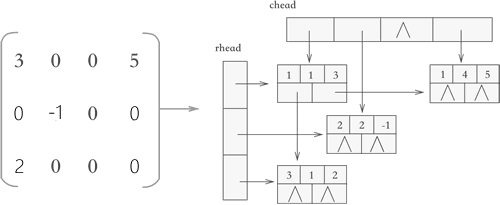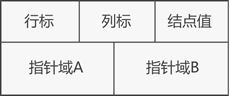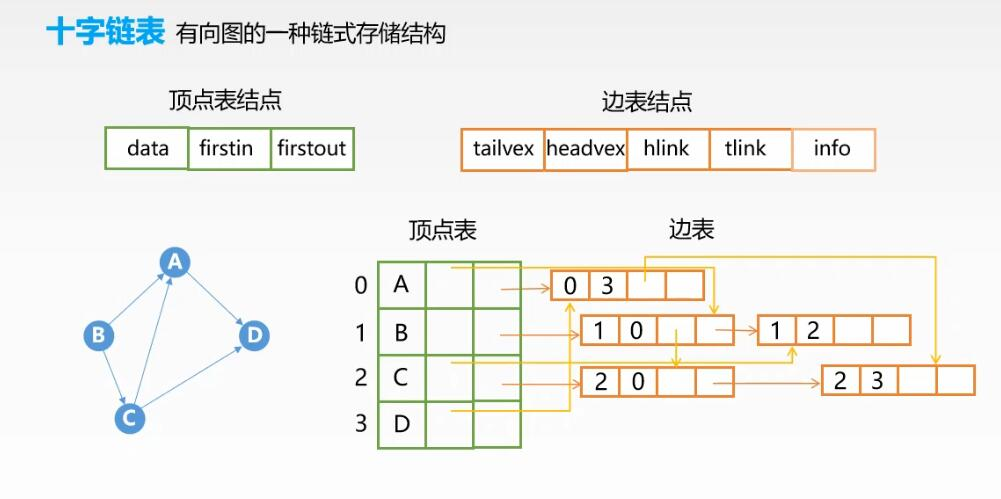• 压缩包中为 十字链表法创建图的 C 文件源文件，及对应的PPT 博客《【经典算法实现 30】图的创建 --- 十字链表法》 链接：https://blog.csdn.net/Ciellee/article/details/108199838
• 对于压缩存储稀疏矩阵，无论是使用三元组顺序，还是使用行逻辑链接的顺序，归根结底是使用数组存储稀疏矩阵。介于数组 "不利于插入和删除数据" 的特点，以上两种压缩存储方式都不适合解决类似 "向矩阵中添加或...
对于压缩存储稀疏矩阵，无论是使用三元组顺序表，还是使用行逻辑链接的顺序表，归根结底是使用数组存储稀疏矩阵。介于数组 "不利于插入和删除数据" 的特点，以上两种压缩存储方式都不适合解决类似 "向矩阵中添加或删除非 0 元素" 的问题。例如，A 和 B 分别为两个矩阵，在实现 "将矩阵 B 加到矩阵 A 上" 的操作时，矩阵 A 中的元素会发生很大的变化，之前的非 0 元素可能变为 0，而 0 元素也可能变为非 0 元素。对于此操作的实现，之前所学的压缩存储方法就显得力不从心。本节将学习用十字链表存储稀疏矩阵，该存储方式采用的是 "链表+数组" 结构，如图 1 所示。图 1 十字链表示意图可以看到，使用十字链表压缩存储稀疏矩阵时，矩阵中的各行各列都各用一各链表存储，与此同时，所有行链表的表头存储到一个数组(rhead)，所有列链表的表头存储到另一个数组(chead)中。因此，各个链表中节点的结构应如图 2 所示:图 2 十字链表的节点结构两个指针域分别用于链接所在行的下一个元素以及所在列的下一个元素。链表中节点的 C 语言代码表示应为：typedef struct OLNode{int i,j;//元素的行标和列标int data;//元素的值struct OLNode * right,*down;//两个指针域}OLNode;同时，表示十字链表结构的 C 语言代码应为：#include#includetypedef struct OLNode{int i, j, e; //矩阵三元组i代表行 j代表列 e代表当前位置的数据struct OLNode *right, *down; //指针域 右指针 下指针}OLNode, *OLink;typedef struct{OLink *rhead, *chead; //行和列链表头指针int mu, nu, tu;  //矩阵的行数,列数和非零元的个数}CrossList;CrossList CreateMatrix_OL(CrossList M);void display(CrossList M);int main(){CrossList M;M.rhead = NULL;M.chead = NULL;M = CreateMatrix_OL(M);printf("输出矩阵M:\n");display(M);return 0;}CrossList CreateMatrix_OL(CrossList M){int m, n, t;int i, j, e;OLNode *p, *q;printf("输入矩阵的行数、列数和非0元素个数：");scanf("%d%d%d", &m, &n, &t);M.mu = m;M.nu = n;M.tu = t;if (!(M.rhead = (OLink*)malloc((m + 1) * sizeof(OLink))) || !(M.chead = (OLink*)malloc((n + 1) * sizeof(OLink)))){printf("初始化矩阵失败");exit(0);}for (i = 1; i <= m; i++){M.rhead[i] = NULL;}for (j = 1; j <= n; j++){M.chead[j] = NULL;}for (scanf("%d%d%d", &i, &j, &e); 0 != i; scanf("%d%d%d", &i, &j, &e)) {if (!(p = (OLNode*)malloc(sizeof(OLNode)))){printf("初始化三元组失败");exit(0);}p->i = i;p->j = j;p->e = e;//链接到行的指定位置if (NULL == M.rhead[i] || M.rhead[i]->j > j){p->right = M.rhead[i];M.rhead[i] = p;}else{for (q = M.rhead[i]; (q->right) && q->right->j < j; q = q->right);p->right = q->right;q->right = p;}//链接到列的指定位置if (NULL == M.chead[j] || M.chead[j]->i > i){p->down = M.chead[j];M.chead[j] = p;}else{for (q = M.chead[j]; (q->down) && q->down->i < i; q = q->down);p->down = q->down;q->down = p;}}return M;}void display(CrossList M) {for (int i = 1; i <= M.nu; i++){if (NULL != M.chead[i]){OLink p = M.chead[i];while (NULL != p){printf("%d\t%d\t%d\n", p->i, p->j, p->e);p = p->down;}}}}运行结果：输入矩阵的行数、列数和非0元素个数：3 3 32 2 32 3 43 2 50 0 0输出矩阵M:2       2       33       2       52       3       4
展开全文• #include<stdio.h>...//十字链表法 存储 稀疏矩阵 //普通节点 typedef struct OLNode{ int col,row; float value; struct OLNode *right,*down; }OLNode; //总头结点–存放：行数，列数，非0元数(k...
#include<stdio.h>
#include<stdlib.h>
#define MAXSIZE 100

//十字链表法 存储 稀疏矩阵

//普通节点
typedef struct OLNode{
int col,row;
float value;
struct OLNode *right,*down;
}OLNode;

//总头结点--存放：行数，列数，非0元数(k)，指向右、下基地址的指针
typedef struct CrossList{
int m,n,k;
}CrossList;

int createCrossListMat(float A[][MAXSIZE],int m,int n,int k,CrossList &M){
//置空指针--可能是第二次调用此函数，所以要置空

M.k=k;
M.m=m;
M.n=n;

//申请基地址空间，没内存则返回
return 0;
return 0;

//基地址置空
for(int i=0;i<n;++i){
}
for(int j=0;j<m;++j){
}

//定义辅助地址，实时更新，指向非零新生结点
OLNode *temp_c[n];
for(int i=0;i<n;++i){
}

//开始存储
for(int i=0;i<m;++i){
//定义辅助地址，实时更新，指向非零新生结点
for(int j=0;j<n;++j){
if(A[i][j]!=0){
OLNode *p=(OLNode*)malloc(sizeof(OLNode));
p->value=A[i][j];
p->row=i;
p->col=j;
p->right=NULL;
p->down=NULL;

temp_c[j]->down=p;
temp_c[j]=p;

r->right=p;
r=p;
}
}
}

return 1;
}

int main(){
return 0;
}




展开全文• 图的存储结构：十字链表法十字链表法的定义：十字链表法的代码定义: 十字链表法的定义： 顶点表节点： 1、data:顶点数据域 2、firstin:入边单链表头指针 3、firstout:出边单链表头指针 边表节点： 1、tailvex:尾域...
图的存储结构：十字链表法思维导图：产生条件：十字链表法的定义：十字链表法的代码定义:性能分析：
思维导图：产生条件：
当用邻接矩阵存储时：空间复杂度为O(|v|^2),太大
当用邻接表法存储时：在进行入度查询时只能进行全部节点遍历，很不方便
十字链表法的定义：
**PS:**只能存储有向图找指定顶点的所有出边：顺着绿色指针找
找指定顶点的所有入边：顺着橙色指针找顶点表节点：
1、data:顶点数据域
2、firstin:入边单链表头指针
3、firstout:出边单链表头指针

边表节点：
1、tailvex:尾域，存放弧尾节点
5、info:权值

十字链表法的代码定义:
#define MaxVertexTypeNum 100
typedef char VertexType;
typedef int EdgeType;

typedef struct ArcNode{				//边表节点
struct ArcNode *hlink , *think;	//出单链表和入单链表
//InfoType info; 				//权值
}ArcNode;							//边表节点的类型

typedef struct VNode{				//顶点表节点
VertexType data;				//顶点的数据
ArcNode *firstin,*firstout;		//入单链表的头指针和入单链表的头指针
}VNode;	// 邻接表类型

typedef struct{						//十字链表
VNode xlist[MaxVertexTypeNum];	//所有结点的数据
int vexnum,arcnum;				//节点数和边数
}ALGraph;							//十字链表的类型

性能分析：
空间复杂度：O(|v| + |E|)


展开全文• 对于压缩存储稀疏矩阵，无论是使用三元组顺序，还是使用行逻辑链接的顺序，归根结底是使用数组存储稀疏矩阵。介于数组 "不利于插入和删除数据" 的特点，以上两种压缩存储方式都不适合解决类似 "...
对于压缩存储稀疏矩阵，无论是使用三元组顺序表，还是使用行逻辑链接的顺序表，归根结底是使用数组存储稀疏矩阵。介于数组 "不利于插入和删除数据" 的特点，以上两种压缩存储方式都不适合解决类似 "向矩阵中添加或删除非 0 元素" 的问题。
例如，A 和 B 分别为两个矩阵，在实现 "将矩阵 B 加到矩阵 A 上" 的操作时，矩阵 A 中的元素会发生很大的变化，之前的非 0 元素可能变为 0，而 0 元素也可能变为非 0 元素。对于此操作的实现，之前所学的压缩存储方法就显得力不从心。
本节将学习用十字链表存储稀疏矩阵，该存储方式采用的是 "链表+数组" 结构，如图 1 所示。图 1 十字链表示意图

因此，各个链表中节点的结构应如图 2 所示:图 2 十字链表的节点结构

两个指针域分别用于链接所在行的下一个元素以及所在列的下一个元素。
链表中节点的 C 语言代码表示应为：

typedef struct OLNode{
int i,j;//元素的行标和列标
int data;//元素的值
struct OLNode * right,*down;//两个指针域
}OLNode;

同时，表示十字链表结构的 C 语言代码应为：

#include<stdio.h>
#include<stdlib.h>
typedef struct OLNode
{
int i, j, e; //矩阵三元组i代表行 j代表列 e代表当前位置的数据
struct OLNode *right, *down; //指针域 右指针 下指针
typedef struct
{
int mu, nu, tu;  //矩阵的行数,列数和非零元的个数
}CrossList;
CrossList CreateMatrix_OL(CrossList M);
void display(CrossList M);
int main()
{
CrossList M;
M = CreateMatrix_OL(M);
printf("输出矩阵M:\n");
display(M);
return 0;
}
CrossList CreateMatrix_OL(CrossList M)
{
int m, n, t;
int i, j, e;
OLNode *p, *q;
printf("输入矩阵的行数、列数和非0元素个数：");
scanf("%d%d%d", &m, &n, &t);
M.mu = m;
M.nu = n;
M.tu = t;
{
printf("初始化矩阵失败");
exit(0);
}
for (i = 1; i <= m; i++)
{
}
for (j = 1; j <= n; j++)
{
}
for (scanf("%d%d%d", &i, &j, &e); 0 != i; scanf("%d%d%d", &i, &j, &e)) {
if (!(p = (OLNode*)malloc(sizeof(OLNode))))
{
printf("初始化三元组失败");
exit(0);
}
p->i = i;
p->j = j;
p->e = e;
//链接到行的指定位置
{
}
else
{
for (q = M.rhead[i]; (q->right) && q->right->j < j; q = q->right);
p->right = q->right;
q->right = p;
}
//链接到列的指定位置
{
}
else
{
for (q = M.chead[j]; (q->down) && q->down->i < i; q = q->down);
p->down = q->down;
q->down = p;
}
}
return M;
}
void display(CrossList M) {
for (int i = 1; i <= M.nu; i++)
{
{
while (NULL != p)
{
printf("%d\t%d\t%d\n", p->i, p->j, p->e);
p = p->down;
}
}
}
}

运行结果：

输入矩阵的行数、列数和非0元素个数：3 3 3
2 2 3
2 3 4
3 2 5
0 0 0
输出矩阵M:
2       2       3
3       2       5
2       3       4


展开全文稀疏矩阵的压缩存储 C语言 数据结构
• 【经典算法实现 30】十字链表实现图的保存一、十字链表法1.1 画出所有顶点1.2 画出所有的边1.3 完善边与顶点的出边关系1.4 完善边与顶点的入边关系二、十字链表代码实现 之前写了这么多树的文章，本文要开始学习图了...链表 指针 算法 数据结构
• 对于压缩存储稀疏矩阵，无论是使用三元组顺序，还是使用行逻辑链接的顺序，归根结底是使用数组存储稀疏矩阵。介于数组 “不利于插入和删除数据” 的特点，以上两种压缩存储方式都不适合解决类似 “向矩阵中添加...链表 数据结构 算法 c语言
• 十字链表法的创建： 1 typedef struct OLNode 2 { 3 int row,col; 4 int value; 5 struct LONode *right,*down; 6 }OLNode,*OLink; 7 8 typedef struct 9 { 10 OLink *row_head,*col_h...
• 文章目录三元组顺序行逻辑链接的顺序表十字链表 三元组顺序   稀疏矩阵由于其自身的稀疏特性，通过压缩可以大大节省稀疏矩阵的内存代价。具体操作是：将非零元素所在的行、列以及它的值构成一个三元组（i，j...稀疏矩阵 数据结构与算法
• ## AOI之十字链表法

千次阅读 2018-05-29 20:35:22
1. 简介AOI主要有九宫格、灯塔和十字链表的算法实现。本文阐述十字链表的实现2. 基本原理若是二维地图，将地图内的对象按照坐标值，从小到大分在x轴和y轴两个链表上。如果是三维地图，则还需要维护多一个z轴的链表3...
• //释放十字链表空间 int main() {  pList L = Init();  int count = 1;  while (count <= L->num)  {  Insert(L);  count++;  }  puts("按行打印三元组");  Ptint1(L);  puts("按列打印三元...
• 【创建】 CrossList* Create(int A[][maxSize], int m, int n) { CrossList* cl = (CrossList*)malloc(sizeof(CrossList)); cl->m = m; cl->n = n; cl->k = 0; ... //申请头结点...
• 个人理解： 可以设想一个场景，一个矩阵是从左往右，从上往下，按左上角到右下角的方向生成的。在每个行和列交叉的节点上存储数据。那这些数据间有何种联系，采用何种方式可以方便的对任意节点的数据进行读写操作？...数据结构
• 文章目录一、十字链表结构体二、十字链表代码 一、十字链表结构体 #define MaxSize 5 //结构体 //头结点 typedef struct{ int m; int n; int k; DLNode *rhead; DLNode *chead; }CrossList; //普通结点 ...数据结构 c语言 矩阵...